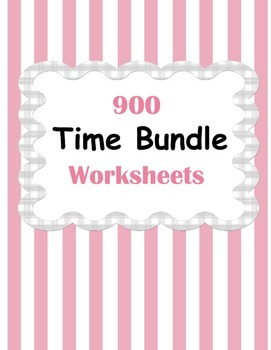Time Worksheets BundleSubject
Resource Type
Product Rating
File Type

Compressed Zip File

49 MB|1,312 pages
Share
Product Description
Time Worksheets Bundle includes these products:
Time Worksheets (What time is it?)
Telling Time
Elapsed Time Worksheets
Time Conversion

When purchased individually, the cost of these products totals \$21.00

Time Worksheets (What time is it?)
What time is it? (12 hours) - 50 Worksheets
What time is it? (24 hours) - 50 Worksheets

Telling Time
Telling Time - Hours. (10 Worksheets)
Telling Time - Half Hourly. (10 Worksheets)
Telling Time - Quarters. (10 Worksheets)
Telling Time - Five Minutes. (10 Worksheets)
Telling Time - Minutes. (10 Worksheets)

Elapsed Time Worksheets
Elapsed Time. Nearest Hour. (20 Worksheets)
Elapsed Time. Nearest Half Hour. (20 Worksheets)
Elapsed Time. Nearest Quarter. (20 Worksheets)
Elapsed Time. Nearest 5 minutes. (20 Worksheets)
Elapsed Time. Nearest minutes. (20 Worksheets)
Start Time. Nearest Hour. (20 Worksheets)
Start Time. Nearest Half Hour. (20 Worksheets)
Start Time. Nearest Quarter. (20 Worksheets)
Start Time. Nearest 5 minutes. (20 Worksheets)
Start Time. Nearest minutes. (20 Worksheets)
End Time. Nearest Hour. (20 Worksheets)
End Time. Nearest Half Hour. (20 Worksheets)
End Time. Nearest Quarter. (20 Worksheets)
End Time. Nearest 5 minutes. (20 Worksheets)
End Time. Nearest minutes. (20 Worksheets)

Time Conversion
Seconds and minutes:
- Convert between seconds (sec) and minutes (min).
- Convert the following to seconds (sec).
- Convert the following to minutes (min) and seconds (sec).
Minutes and hours:
- Convert between minutes (min) and hours (hr).
- Convert the following to minutes (min).
- Convert the following to hours (hr) and minutes (min).
Hours and days:
- Convert between hours (hr) and days (d).
- Convert the following to hours (hr).
- Convert the following to days (d) and hours (hr).
Each topic contains 30 Worksheets!

Adding minutes and seconds. (30 Worksheets)
Adding hours and minutes. (30 Worksheets)
Adding days and hours. (30 Worksheets)
Subtracting minutes and seconds. (30 Worksheets)
Subtracting hours and minutes. (30 Worksheets)
Subtracting days and hours. (30 Worksheets)
Total Pages
1,312 pages
N/A
Teaching Duration
N/A
Report this Resource\$13.00